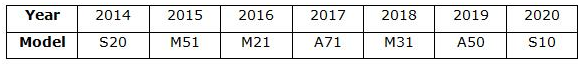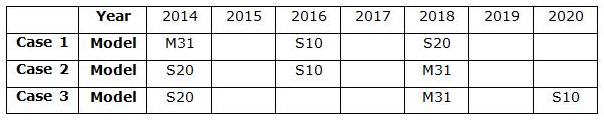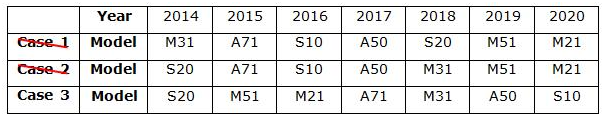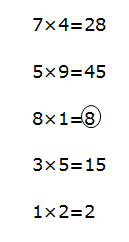# SBI Clerk Prelims Reasoning Ability Questions 2021 (Day-60)

Dear Aspirants, Our IBPS Guide team is providing new series of Reasoning Questions for SBI Clerk Prelims 2021 so the aspirants can practice it on a daily basis. These questions are framed by our skilled experts after understanding your needs thoroughly. Aspirants can practice these new series questions daily to familiarize with the exact exam pattern and make your preparation effective.

Start Quiz

Year puzzle

Direction (1-5): Answer the questions based on the information given below.

Samsung galaxy has launched seven different mobile models viz. A50, A71, M21, M31, M51, S10 and S20 in every year starting from 2014 to 2020, but not necessarily in the same order. Not more than one model was launched in the same year.

S10 was launched in a leap year and two years away from M31. Only three models were launched between M31 and S20.M51 was launched immediately before M21 which was launched in an even year. A50 was launched after A71 and in a non-prime numbered year.

1) Which of the following model was launched in 2015?

A.A50

B.A71

C.M51

D.M31

E.None of these

2) How many mobile models were launched between M51 and S10?

A.One

B.Two

C.Three

D.More than three

E.None

3) In which of the following year does A71 was launched?

A.2015

B.2017

C.2018

D.2016

E.2019

4) If all the mobile models are launched in alphabetical and ascending order, then the position of how many models remains unchanged?

A.One

B.Two

C.Three

D.More than three

E.None

5) Four of the following five are alike in a certain way and hence form a group. Find the one that doesn’t belong to that group.

A.M51

B.M31

C.A50

D.S20

E.S10

Inequality

Direction (6-8): In each of the following questions, the relationship between different elements is shown in the statements followed by two conclusions. Find a conclusion which logically follows.

6) Statements:

B < G ≤ L = V > R = T > M

Conclusions:

I) B < V

II) L > M

A.If only conclusion I follows.

B.If only conclusion II follows.

C.If either conclusion I or II follows.

D.If neither conclusion I nor II follows.

E.If both conclusions I and II follow

7) Statements:

T ≤ X < K = Z > R < P < O

Conclusions:

I)T < Z

II)K < O

A.If only conclusion I follows

B.If only conclusion II follows

C.If either conclusion I or II follows

D.If neither conclusion I nor II follows

E.If both conclusions I and II follows

8) Statements:

M < X ≤ N < V=J > O ≥ G > I

Conclusions:

I)M < J

II)V ≥ I

A.If only conclusion I follows.

B.If only conclusion II follows

C.If either conclusion I or II follows

D.If neither conclusion I nor II follows

E.If both conclusions I and II follows

Number series

Direction (9-10): Study the following information carefully and answer the question below.

724   539   861   375   192

9) If 1 is subtracted from the 2nd digit of each number then what is the sum of the lowest digit of 2nd highest number and the highest digit of the lowest number?

A.9

B.8

C.6

D.10

E.14

10) If 1st and 3rddigits of each number are multiplied, then what is the 2nd lowest value obtained?

A.15

B.8

C.21

D.27

E.18

Directions (1-5) :1. From the given statements,S10 was launched in a leap year and two years away from M31. Only three models were launched between M31 and S20.So, we have three possible cases i.e. case 1, case 2 and case 3:2. M51 was launched immediately before M21 which was launched in an even year. A50 was launched after A71 and in a non-prime numbered year. Here, 2017 is a prime numbered year. Hence, case 1 and 2 get eliminated.724  539  861  375   192

714  529  851  365  182

Lowest digit of the 2nd highest number = 1

Highest digit of the lowest number= 8

8+1=9